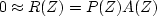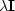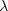Next: Zapping the null space Up: 2-D INTERPOLATION BEYOND ALIASING Previous: The prediction form of

## The regression codes

The programs for the two-dimensional prediction-error filter and missing data resemble those for one dimension. I simplified the code by not trying to pack the unknowns and residuals tightly in the abstract vectors. Because of this, it is necessary to be sure those abstract vectors are initialized to zero. (Otherwise, the parts of the abstract vector that are not initialized could contribute to the result when cgstep()evaluates dot products on abstract vectors.) The routine pe2()finds the 2-D PE filter.
# Find spatial prediction-error filter.
#
subroutine pe2( eps, a1,a2,aa, n1,n2 ,pp, rr, niter, jump)
integer              a1,a2,    n1,n2,         niter, jump
integer i1, iter, midpt, r12, a12
real    aa( a1 , a2),  pp( n1 , n2),  rr( n1 , n2 * 2),  eps
temporary real da( a1, a2),  dr( n1, n2 * 2)
temporary real sa( a1, a2),  sr( n1, n2 * 2)
r12 = n1 * n2
a12 = a1 * a2
call null( aa, a12);    call null( rr, 2 * r12)
call null( da, a12);    call null( dr, 2 * r12)
midpt = (a1+1) / 2
aa( midpt, 1 ) = 1.
call cinjof( 0, 0, jump, n1,n2,pp, a1,a2,aa, rr         )
call ident ( 0, 0, eps,            a12,  aa, rr(1,n2+1) )
call scaleit (       -1.,          2*r12,      rr         )
do iter= 0, niter {
call cinjof( 1, 0, jump, n1,n2,pp, a1,a2,da, rr         )
call ident ( 1, 1,  eps,           a12,  da, rr(1,n2+1) )
do i1= 1, a1 {                           da(i1, 1) = 0. }
call cinjof( 0, 0, jump, n1,n2,pp, a1,a2,da, dr         )
call ident ( 0, 0,  eps,           a12,  da, dr(1,n2+1) )
call cgstep( iter,   a12, aa,da,sa, _
2*r12, rr,dr,sr )
}
return; end


This routine is the two-dimensional equivalent of finding the filter A(Z) so that.We coded the 1-D problem in iner(). In pe2(), however, I did not bother with the weighting functions. A further new feature of pe2() is that I addedcapability (whereis eps) by including the call to ident(), so that I could experiment with various forms of filter stabilization. (This addition did not seem to be helpful.)

Given the 2-D PE filter, the missing data is found with miss2(), which is the 2-D equivalent of miss1().

# fill in missing data in 2-D by minimizing power out of a given filter.
#
subroutine miss2(   lag1,lag2, a1,a2, aa, n1,n2, ww, pp, known, rr, niter)
integer i1,i2,iter, lag1,lag2, a1,a2,     n1,n2,                    niter, n12
real    pp(    n1, n2)          # in: known data with zeros for missing values
# out: known plus missing data.
real    known( n1, n2)          # in: known(ip) vanishes where pp(ip) is missing
real    ww(    n1, n2)          # in: weighting function on data pp
real    aa(    a1, a2)          # in: roughening filter
real    rr(    n1, n2*2)        # out: residual
temporary real  dp( n1, n2), dr( n1, n2*2)
temporary real  sp( n1, n2), sr( n1, n2*2)
n12 = n1 * n2;          call null( rr, n12*2)
call null( dp, n12);    call null( dr, n12*2)
call cinloi( 0, 0, lag1,lag2,a1,a2,aa, n1,n2, pp, rr        )
call diag  ( 0, 0,                 ww,  n12,  pp, rr(1,n2+1))
call scaleit (-1.,                      2*n12,      rr        )
do iter= 0, niter {
call cinloi( 1, 0, lag1,lag2,a1,a2,aa, n1,n2, dp, rr        )
call diag  ( 1, 1,                 ww,  n12,  dp, rr(1,n2+1))
do i1= 1, n1 {
do i2= 1, n2 {  if( known(i1,i2) != 0.)       dp(i1,i2) = 0.
}}
call cinloi( 0, 0, lag1,lag2,a1,a2,aa, n1,n2, dp, dr        )
call diag  ( 0, 0,                 ww,  n12,  dp, dr(1,n2+1))
call cgstep( iter,   n12, pp,dp,sp, _
2*n12, rr,dr,sr )
}
return; end

We will soon see that stabilization is more critical in miss2() than in pe2(). Furthermore, miss2() must be stabilized with a weighting function, here ww(,), which is why I used the diagonal matrix multiplier diag() rather than the identity matrix I used in deghost()and pe2(). Subroutine diag() is used so frequently that I coded it in a special way to allow the input and output to overlie one another.
subroutine diag( adj, add, lambda,n,  pp,    qq)
real                       lambda(n), pp(n), qq(n)
if( adj == 0 ) {
if( add == 0 ) { do i=1,n {  qq(i) =         lambda(i) * pp(i) } }
else           { do i=1,n {  qq(i) = qq(i) + lambda(i) * pp(i) } }
}
else {  if( add == 0 ) { do i=1,n {  pp(i) =         lambda(i) * qq(i) } }
else           { do i=1,n {  pp(i) = pp(i) + lambda(i) * qq(i) } }
}
return; endNext: Zapping the null space Up: 2-D INTERPOLATION BEYOND ALIASING Previous: The prediction form of
Stanford Exploration Project
10/21/1998